# Everything about RSI - page 61352

Hi Mladen,

May be can you find some idea in this wonderful indicator of Petr, with auto-trendline and LRC

May be...

Thanks a lot and have a nice day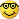Zilliq

divergence_petr.mq4

Files:216393

zilliq:
Hi Mladen,

May be can you find some idea in this wonderful indicator of Petr, with auto-trendline and LRC

May be...

Thanks a lot and have a nice dayZilliq

divergence_petr.mq4

Zilliq

None of those objects in that indicator is a LRC. This is a LRC on RSI : https://www.mql5.com/en/forum/general but in the way you are proposing it, it (linear regression channel) needs to be recalculated for each and every bar and that is not an efficient way of doing it352

Ok thanks a lot Mladen,

I hoped so

Have a very nice day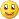Zilliq216393

zilliq:
Ok thanks a lot Mladen,

I hoped so

Have a very nice dayZilliq

Zilliq

This is (as an example) a multiple output function that calculates linear regression line value, slope and standard error which are all needed to calculate linear regression channel :

//------------------------------------------------------------------

//

//------------------------------------------------------------------

//

//

//

//

//

double workLr[];

void fillLrArray(int i, int instanceNo, double value)

{

if (ArrayRange(workLr,0)!=Bars) ArrayResize(workLr,Bars); i = Bars-i-1; workLr = value;

}

double iLrValue(double value, int period, double& slope, double& error, int r, int instanceNo=0)

{

if (ArrayRange(workLr,0)!=Bars) ArrayResize(workLr,Bars); r = Bars-r-1; workLr[r] = value;

if (r<period || period<2) return(value);

//

//

//

//

//

double sumx=0, sumxx=0, sumxy=0, sumy=0, sumyy=0;

for (int k=0; k<period; k++)

{

double price = workLr[r-k];

sumx += k;

sumxx += k*k;

sumxy += k*price;

sumy += price;

sumyy += price*price;

}

slope = (period*sumxy-sumx*sumy)/(sumx*sumx-period*sumxx);

error = MathSqrt((period*sumyy-sumy*sumy-slope*slope*(period*sumxx-sumx*sumx))/(period*(period-2)));

//

//

//

//

//

return((sumy + slope*sumx)/period);

}

All the needs to be calculated in order to enable us to draw a linear regression channel using rsi values.

I did think that there is some way that existing linear regression object can be used for that, but all my attempts to use that object failed and the resulting channel was never appropriate. So the only way (as far as I see it since I never found what is the third set of time+price in the object create function for linear regression channel used for) is to always calculate linear regression values for variable lengths (since the leg of the zigzag is determining the linear regression calculating length) and that is, using mql, putting a great burden on a CPU (tested that too and it is much more CPU intensive than one would assume at the first impression)352

Thanks a lot Mladen,

It's very nice to you to try to find a solution even if I understand that is seems to be difficult

Not sure to well understand : What is difficult is to draw successive LRC with variable lenght based on the legs of zigzag, or even to draw only the previous LRC based on the previous zigzag is difficult ? Because, in fact we only need the previous LRC based on zigzag, and watch when the RSI break his extension

I tried something to simplify this, but it doesn't work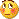I tried to see if we can have some good signals not with the LRC, but with a trendline based on the zigzag

But, as you see it doesn't work, the signals is good, but very late compare to the signal with LRC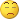I don't know how I can help you

What i'm sure (because I use this system on my plateform), is that the LRC must be based on the zigzag (who repaint or not as I said before)

But if it's easy to say, it's difficult to code as I understand

Have a nice evening, and hope you will find a solution as a challenge for you

Zilliq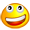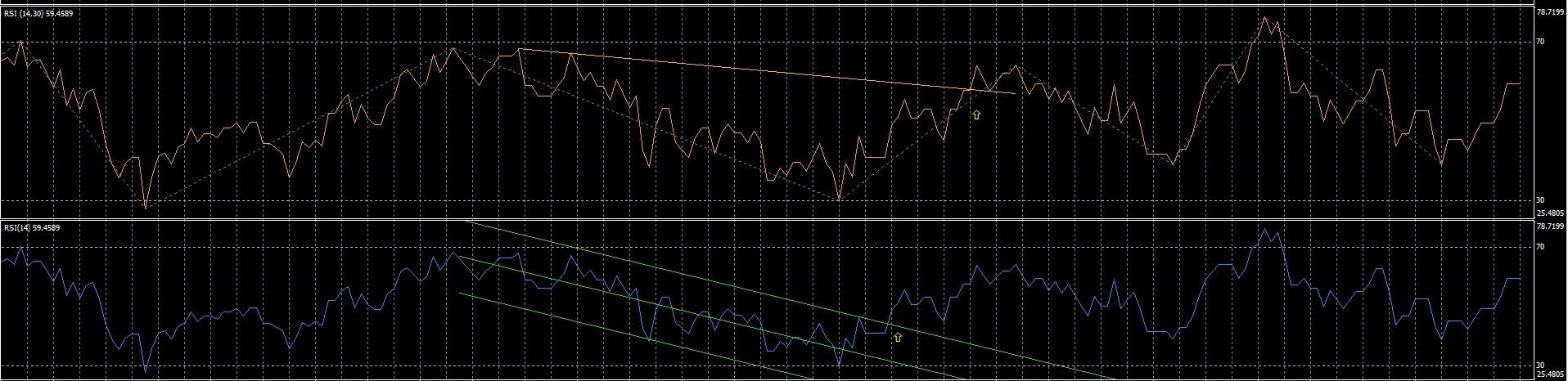Files:2047

Fan RSI indicator (by Alexander Gettinger)

Files:
fan_rsi.png  48 kb
fan_rsi.mq4  3 kb346

pls am looking for rsi cross over with arrow, anyone help me9557

drunkpips:
pls am looking for rsi cross over with arrow, anyone help me

Drunkpips rsi crossing a moving average or rsi crossing oversold/overbought?346

3 different rsi crossing each other and also crossing zero9557

drunkpips:
3 different rsi crossing each other and also crossing zero

Drunkpips, just guessing you meant crossing 50, that's how i made this one.

Files:
rsi_x3.png  51 kb